Non-linear hypothesis, neurons and the brain, model representation, and multi-class classification.

## 1. Motivations

I would like to give full credits to the respective authors as these are my personal python notebooks taken from deep learning courses from Andrew Ng, Data School and Udemy :) This is a simple python notebook hosted generously through Github Pages that is on my main personal notes repository on https://github.com/ritchieng/ritchieng.github.io. They are meant for my personal review but I have open-source my repository of personal notes as a lot of people found it useful.

### 1a. Non-linear Hypothesis

• You can add more features
• But it will be slow to process
• If you have an image with 50 x 50 pixels (greyscale, not RGB)
• n = 50 x 50 = 2500
• quadratic features = (2500 x 2500) / 2
•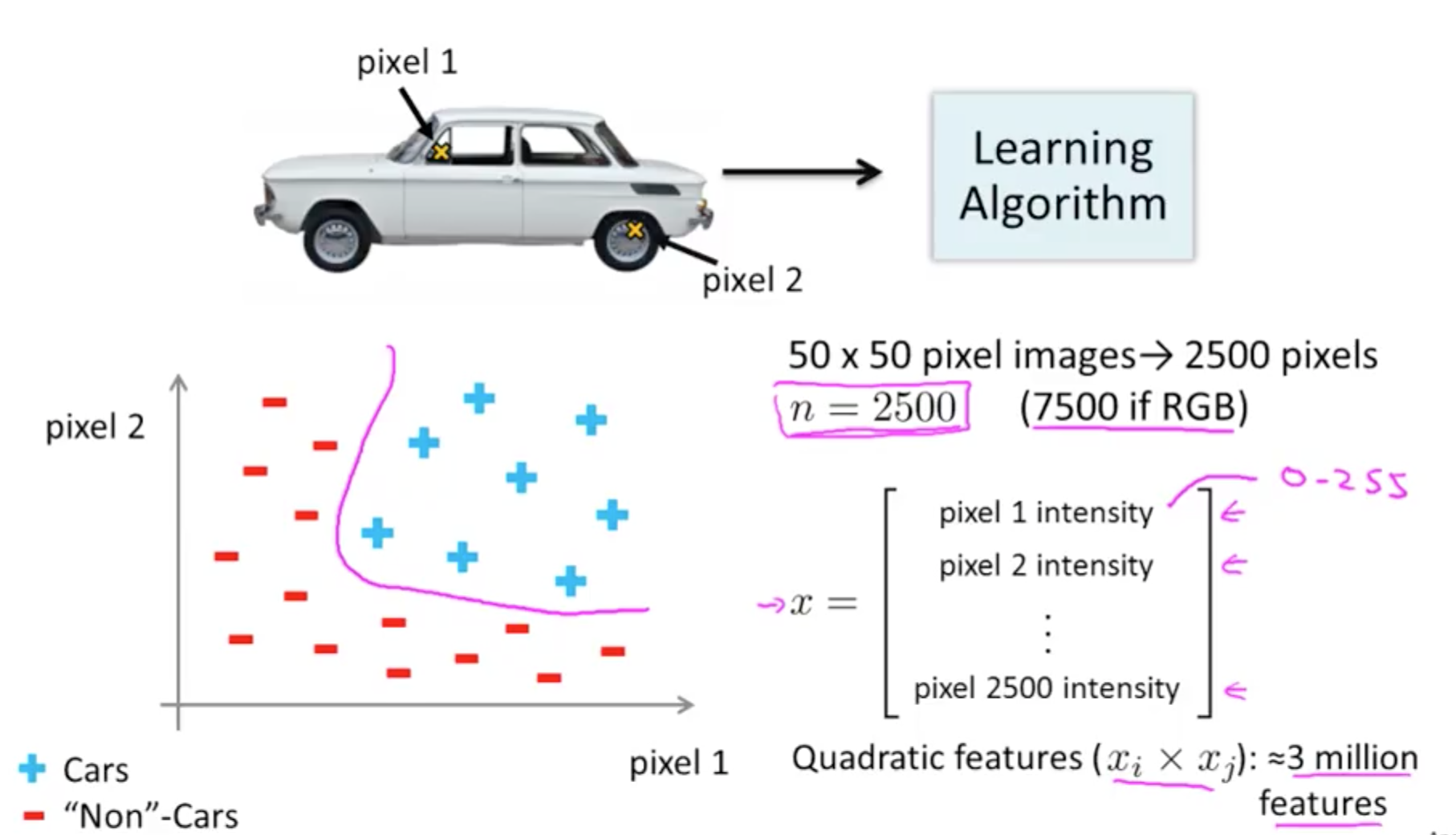• Neural networks are much better for a complex nonlinear hypothesis

### 1b. Neurons and the Brain

• Origins
• Algorithms that try to mimic the brain
• Was very widely used in the 80s and early 90’s
• Popularity diminished in the late 90’s
• Recent resurgence
• State-of-the-art techniques for many applications
• The “one learning algorithm” hypothesis
• Auditory cortex handles hearing
• Re-wire to learn to see
• Somatosensory cortex handles feeling
• Re-wire to learn to see
• Plug in data and the brain will learn accordingly
• Examples of learning
•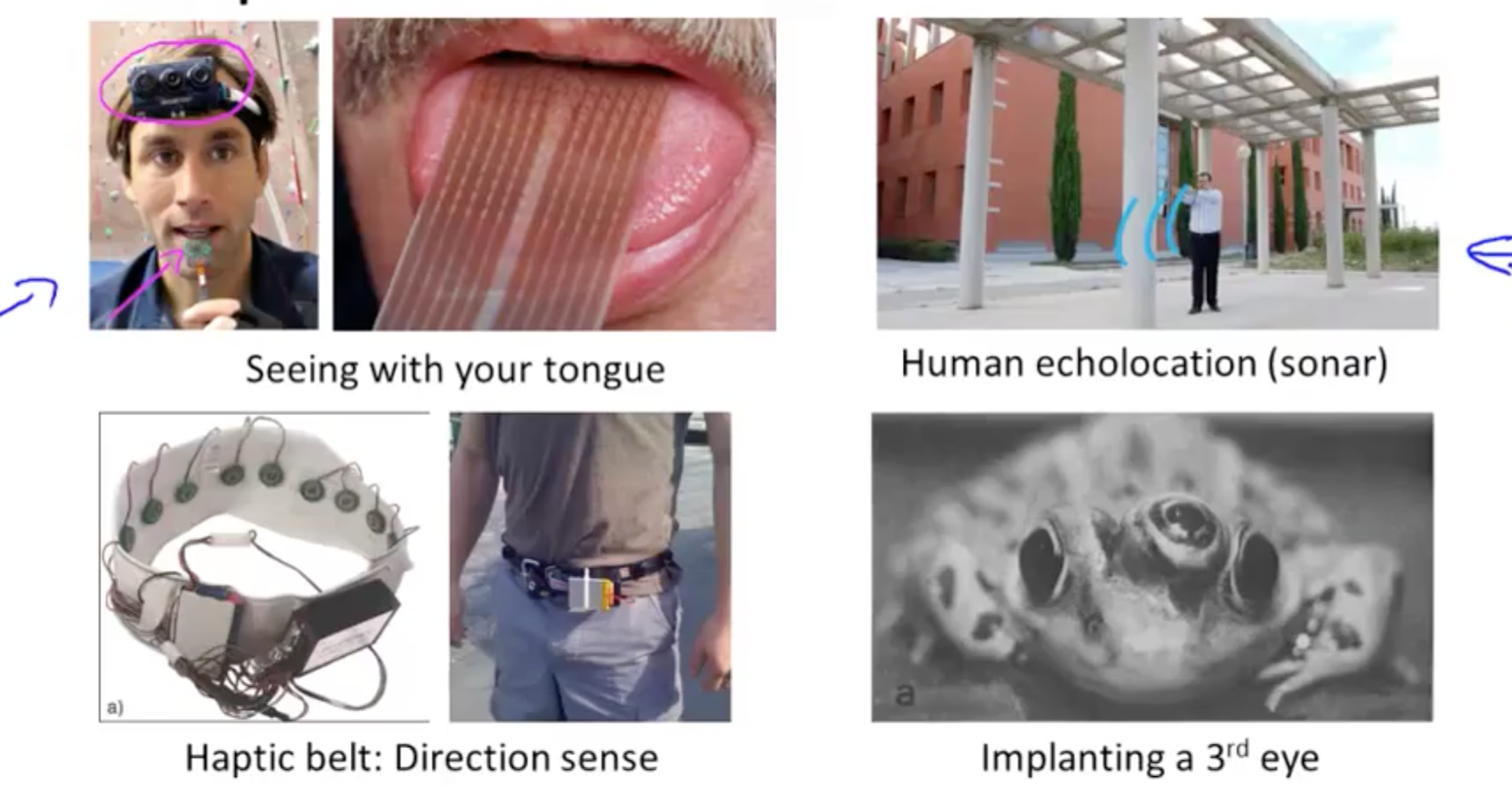## 2. Neural Networks

### 2a. Model Representation I

• Neuron in the brain
• Many neurons in our brain
• Axon: produce output
• When it sends a message through the Axon to another neuron
• It sends to another neuron’s Dendrite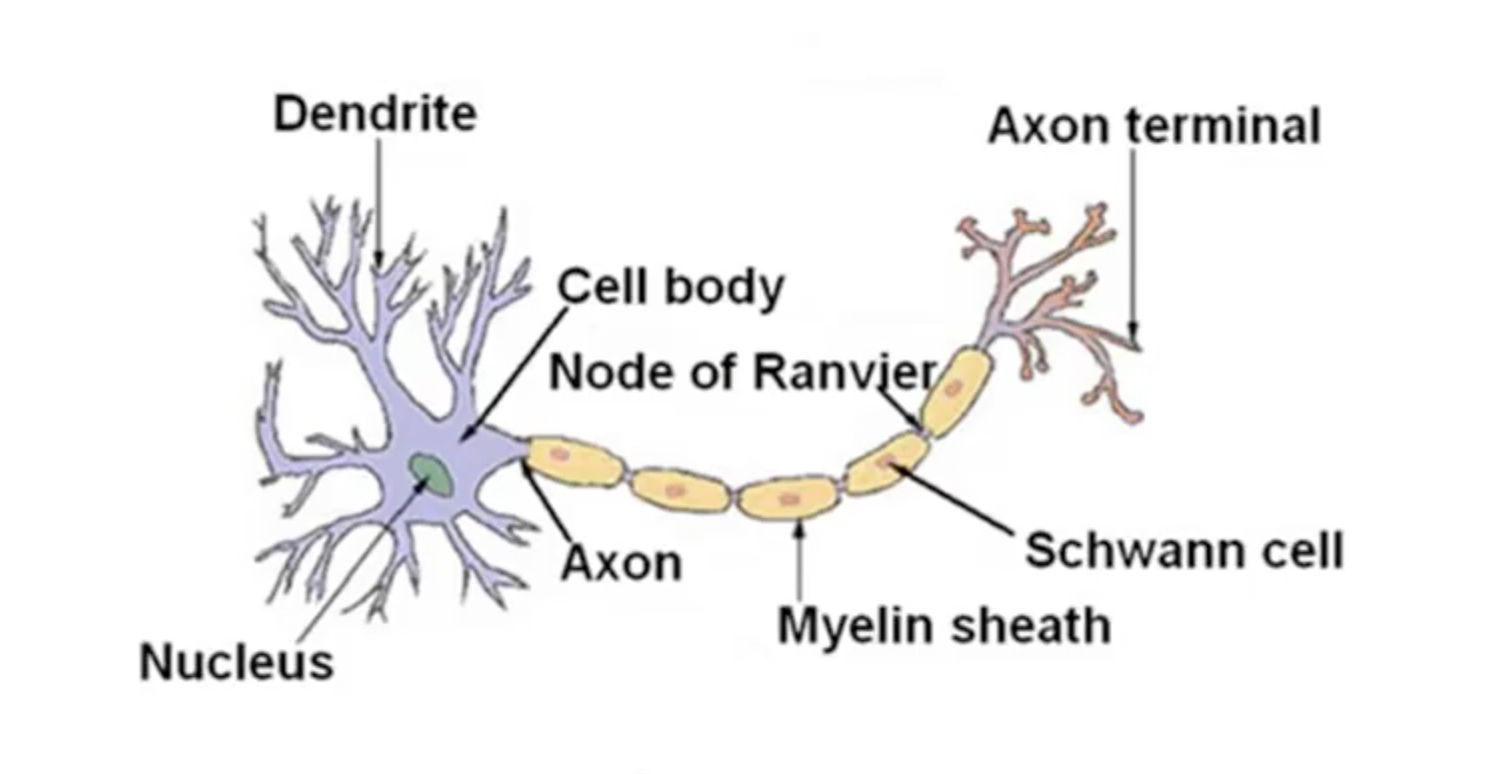• Neuron model: logistic unit
• Yellow circle: body of neuron
• Input wires: dendrites
• Output wire: axon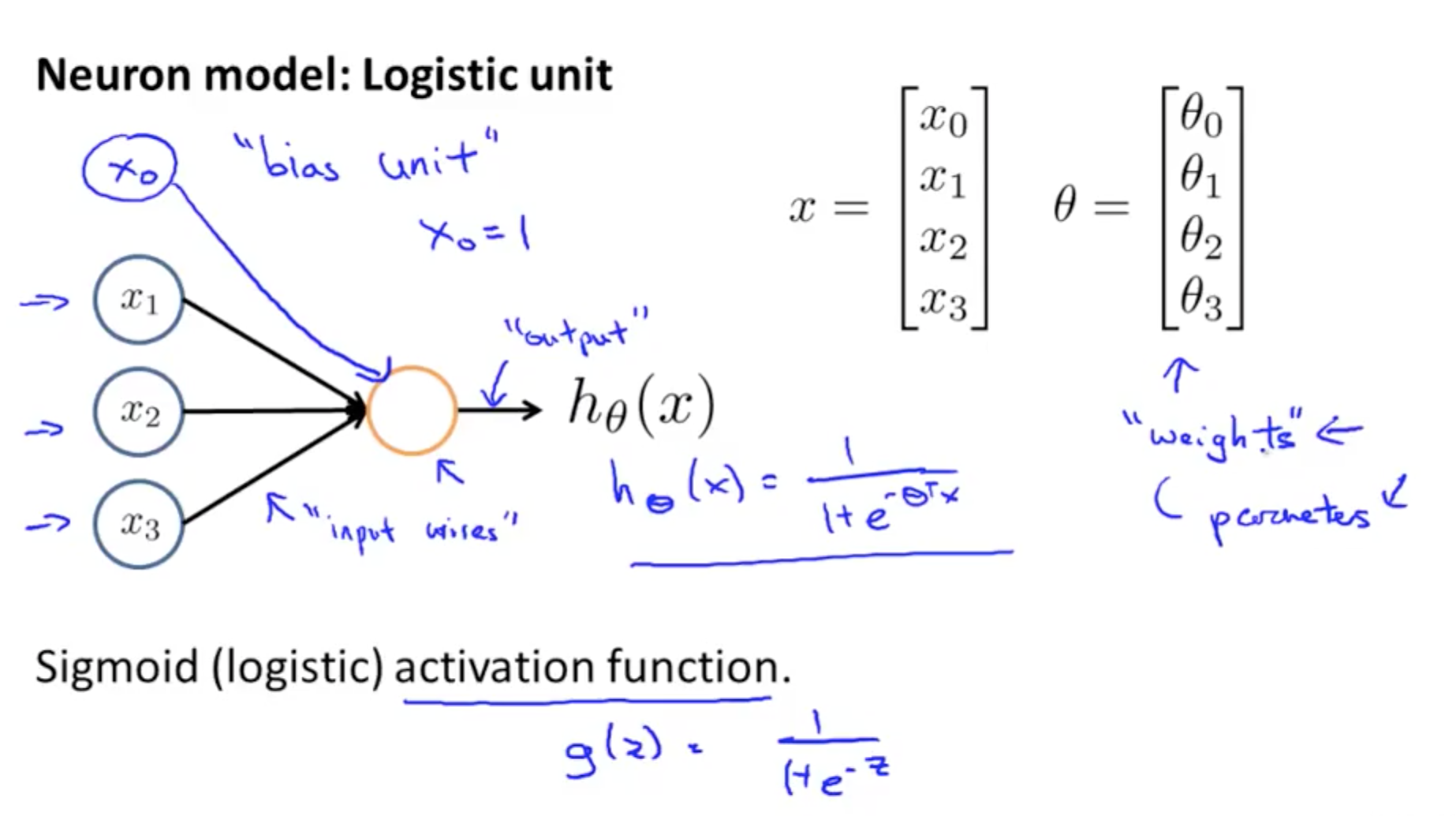• Neural Network
• 3 Layers
• 1 Layer: input layer
• 2 Layer: hidden layer
• Unable to observe values
• Anything other than input or output layer
• 3 Layer: output layer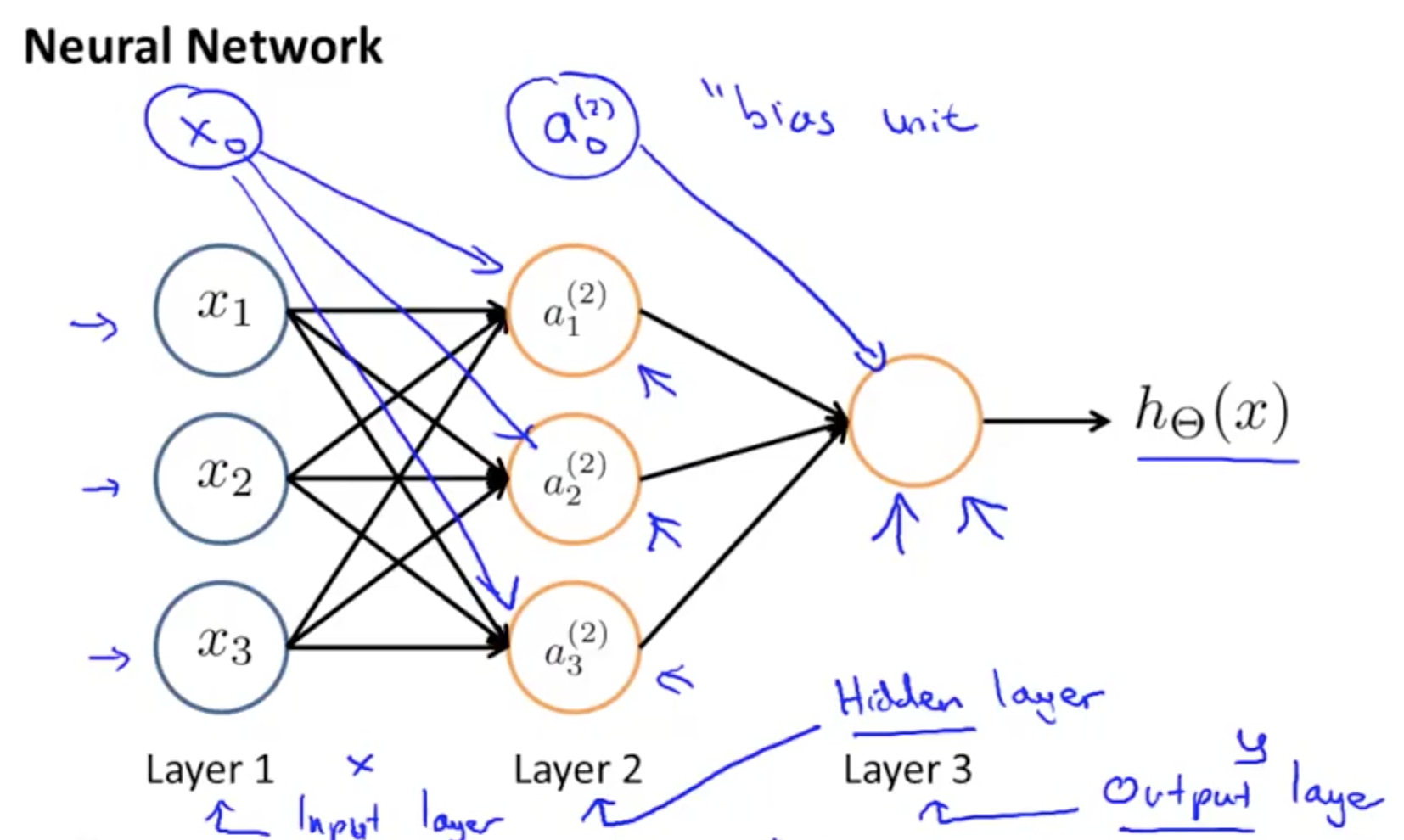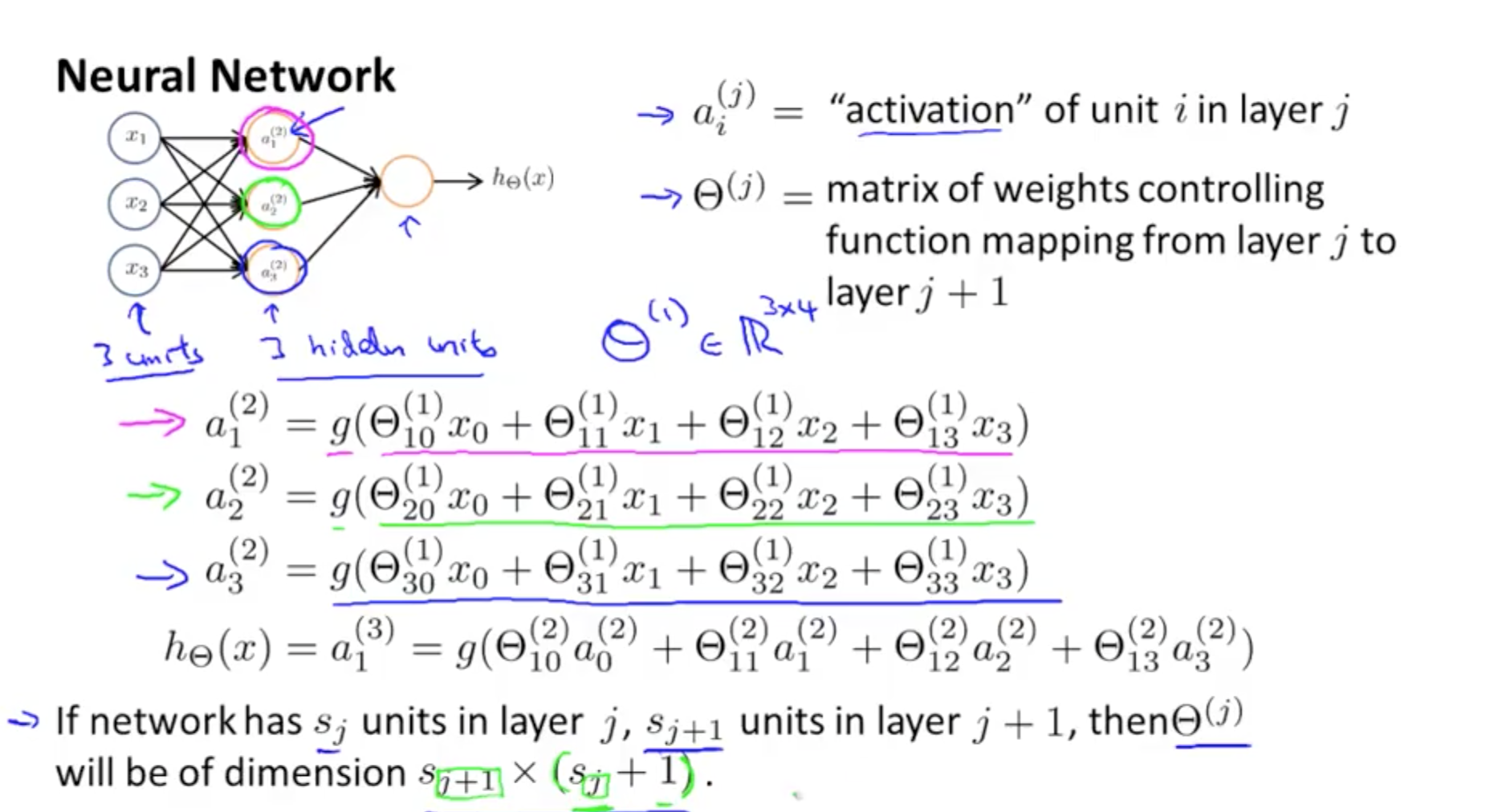• We calculate each of the layer-2 activations based on the input values with the bias term (which is equal to 1)
• i.e. x0 to x3
• We then calculate the final hypothesis (i.e. the single node in layer 3) using exactly the same logic, except in input is not x values, but the activation values from the preceding layer
• The activation value on each hidden unit (e.g. a12 ) is equal to the sigmoid function applied to the linear combination of inputs
• Three input units
• Ɵ(1) is the matrix of parameters governing the mapping of the input units to hidden units
• Ɵ(1) here is a [3 x 4] dimensional matrix
• Three hidden units
• Then Ɵ(2) is the matrix of parameters governing the mapping of the hidden layer to the output layer
• Ɵ(2) here is a [1 x 4] dimensional matrix (i.e. a row vector)
• Every input/activation goes to every node in following layer
• Which means each “layer transition” uses a matrix of parameters with the following significance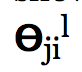• j (first of two subscript numbers)= ranges from 1 to the number of units in layer l+1
• i (second of two subscript numbers) = ranges from 0 to the number of units in layer l
• l is the layer you’re moving FROM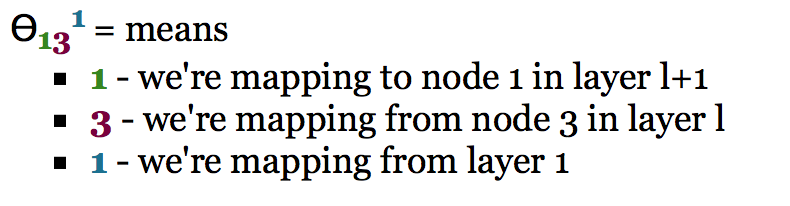• Notation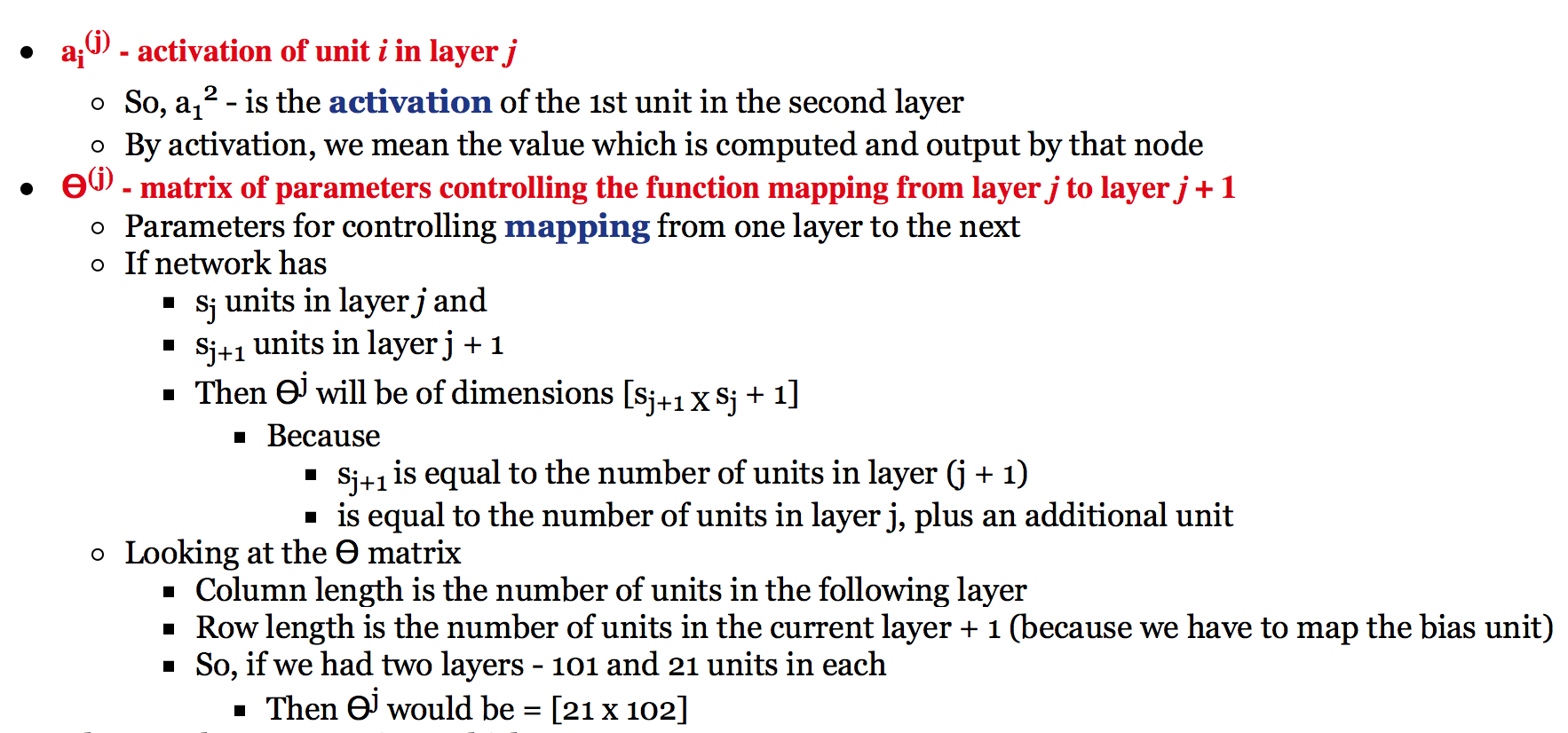### 2a. Model Representation II

• Here we’ll look at how to carry out the computation efficiently through a vectorized implementation. We’ll also consider why neural networks are good and how we can use them to learn complex non-linear things
• Forward propagation: vectorized implementation
• g applies sigmoid-function element-wise to z
• This process of calculating H(x) is called forward propagation
• Worked out from the first layer
• Starts off with activations of input unit
• Propagate forward and calculate the activation of each layer sequentially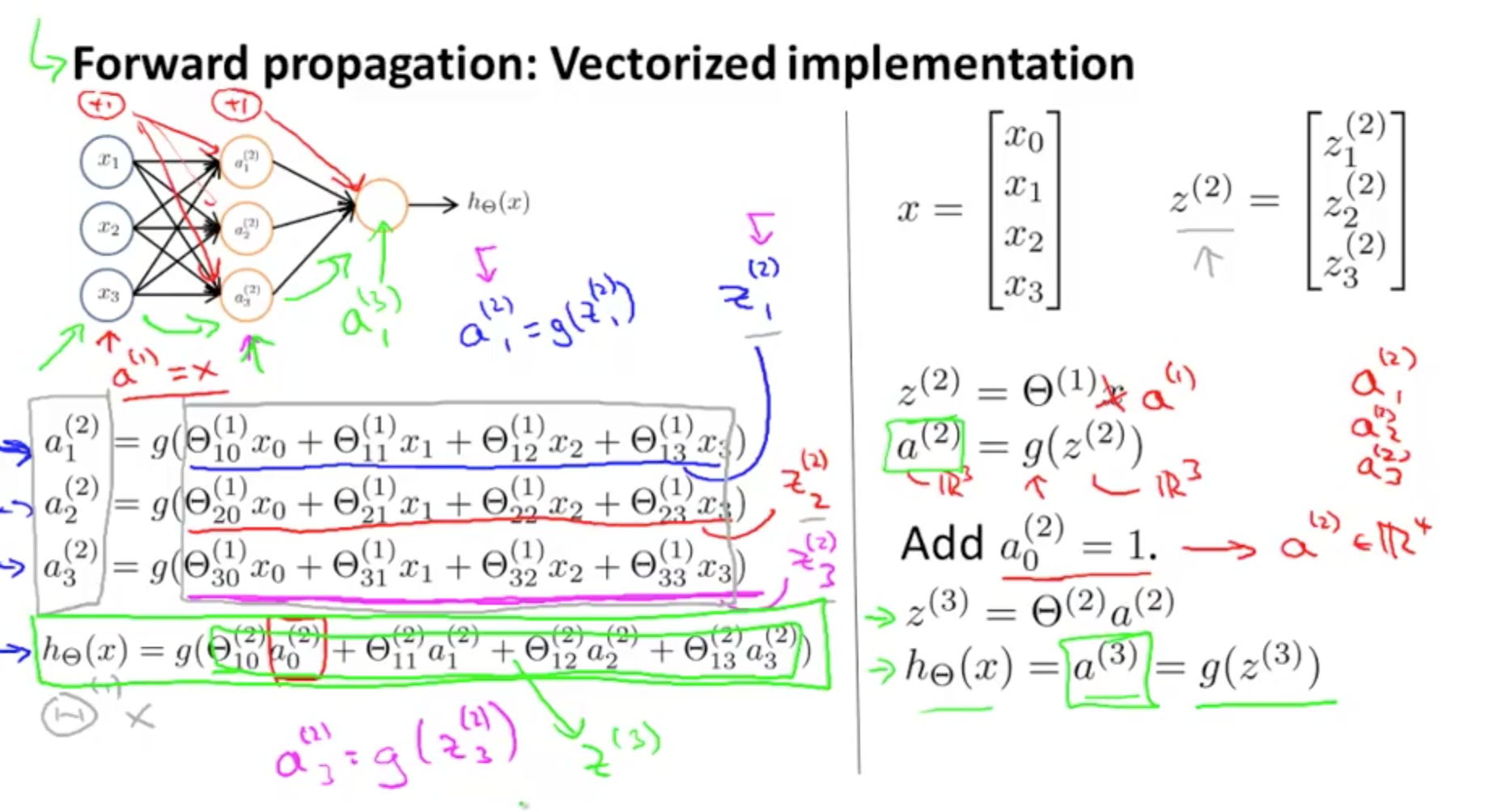• Similar to logistic regression if you leave out the first layer
• Only second and third layer
• Third layer resembles a logistic regression node
• The features in layer 2 are calculated/learned, not original features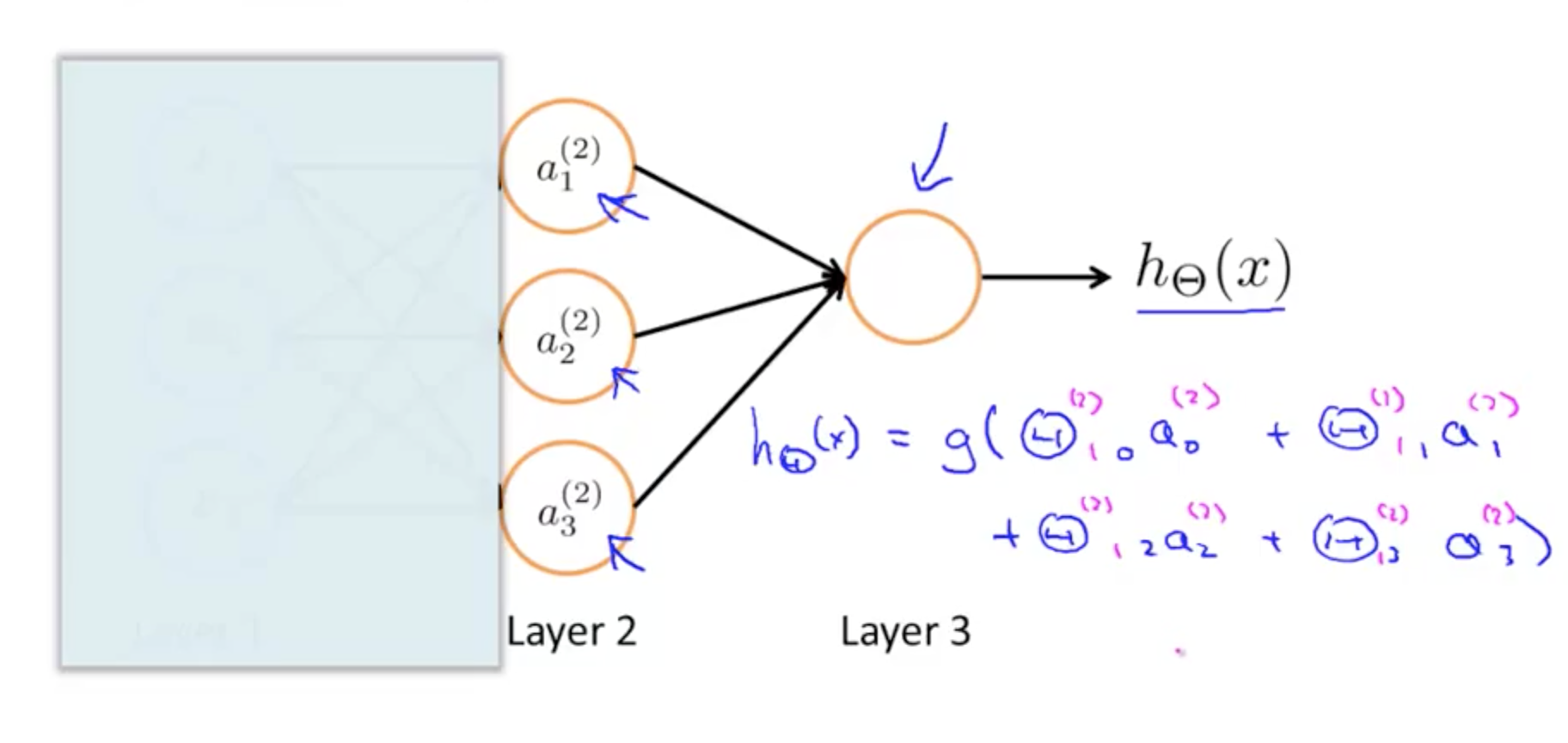• Neural network, learns its own features
• The features a’s are learned from x’s
• It learns its own features to feed into logistic regression
• Better hypothesis than if we were constrained with just x1, x2, x3
• We can have whatever features we want to feed to the final logistic regression function
• Implemention in Octave for a2
• a2 = sigmoid (Theta1 * x);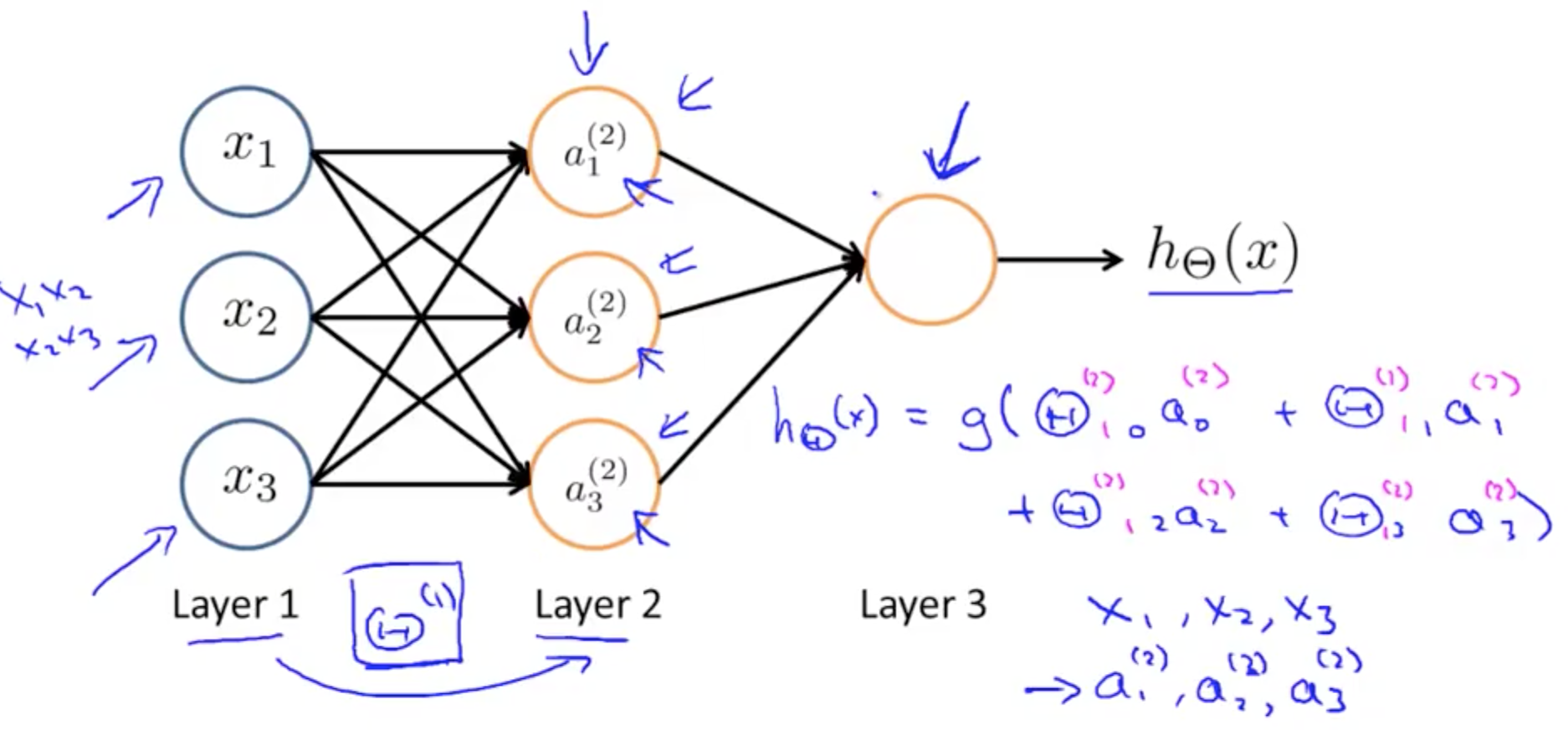• Other network architectures
• Layer 2 and 3 are hidden layers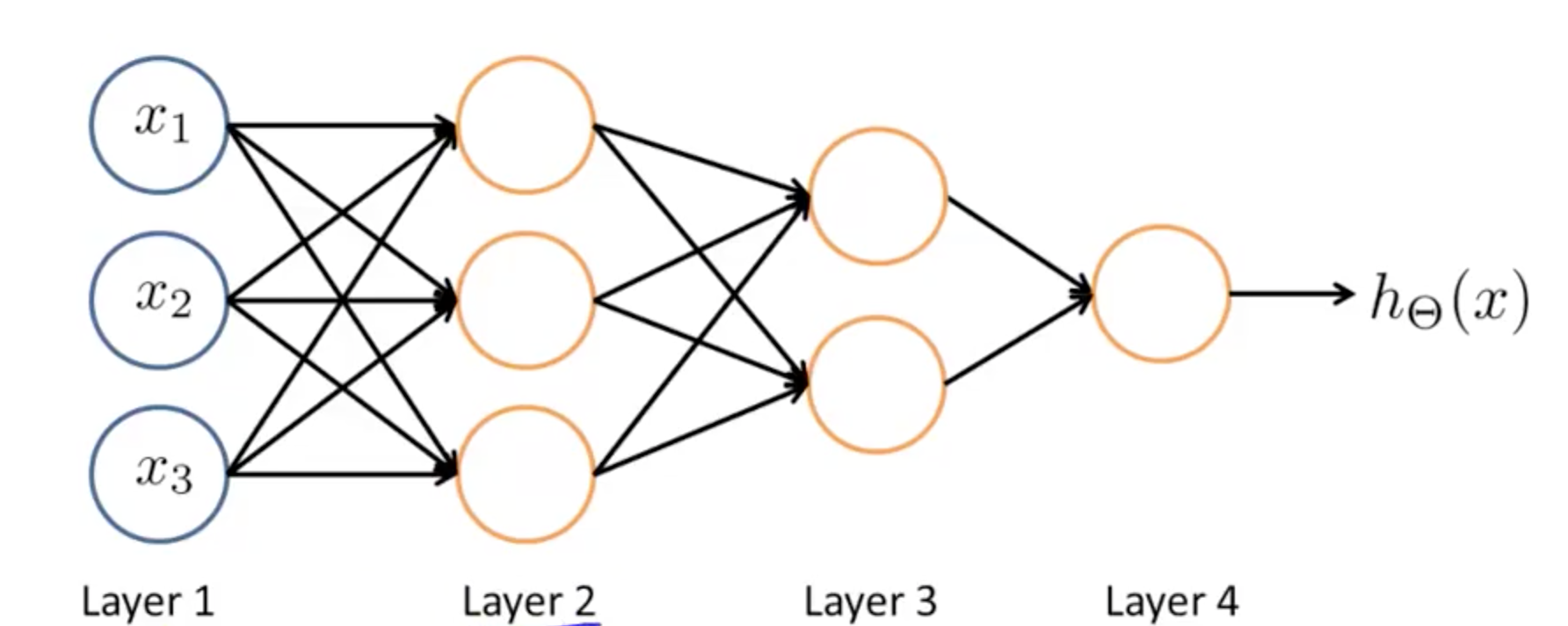## 2. Neural Network Application

### 2a. Examples and Intuitions I

• XOR/XNOR
• XOR: or
• XNOR: not or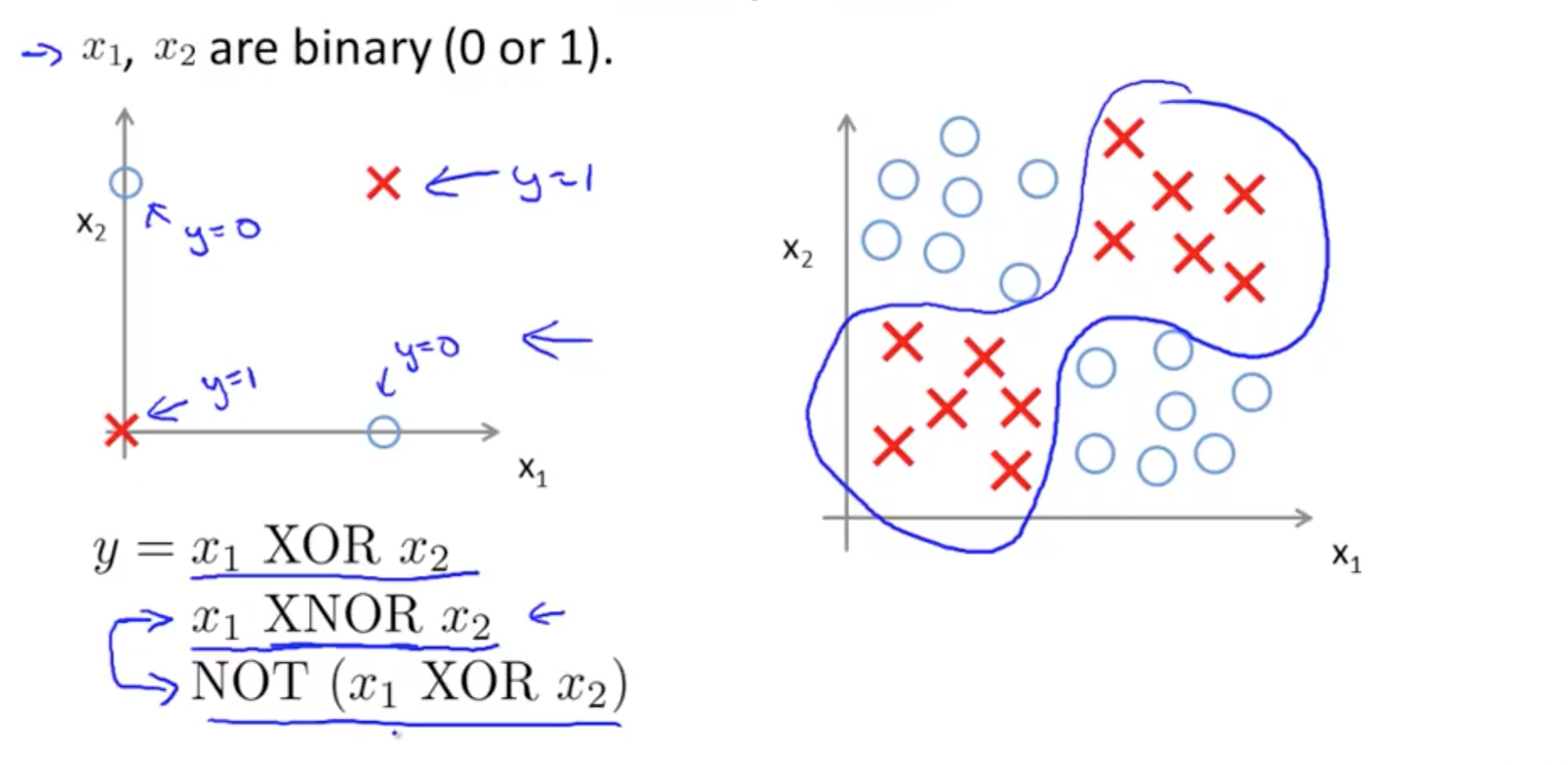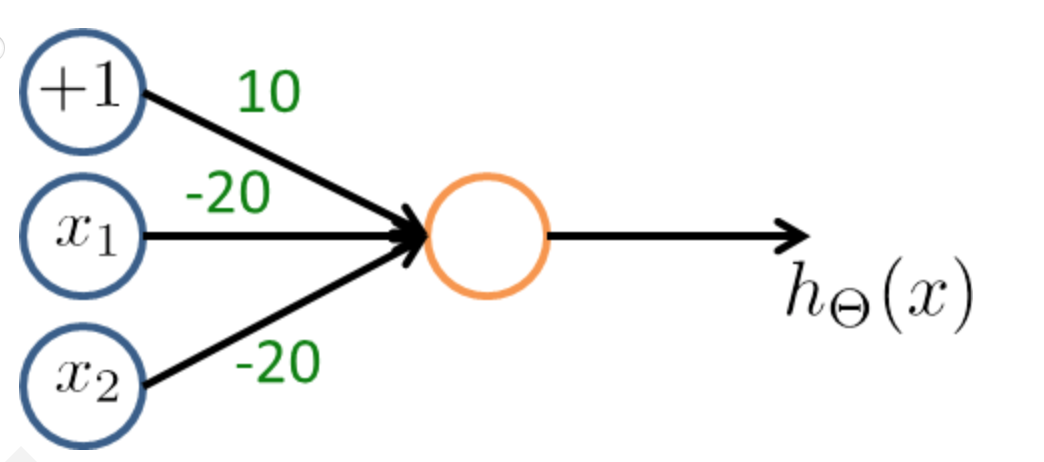• AND function
• Outputs 1 only if x1 and x2 are 1
• Draw a table to determine if OR or AND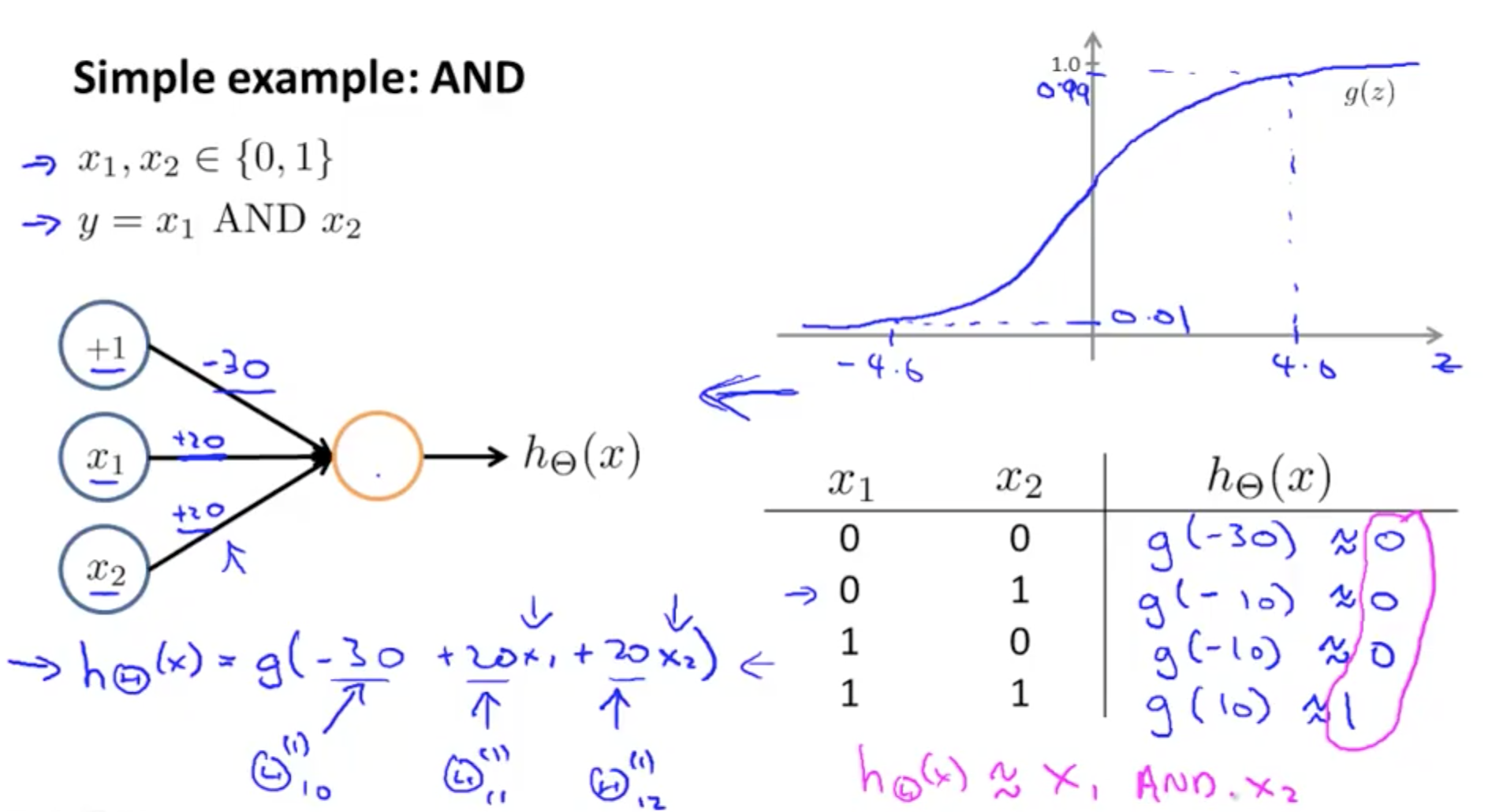• NAND function
• NOT AND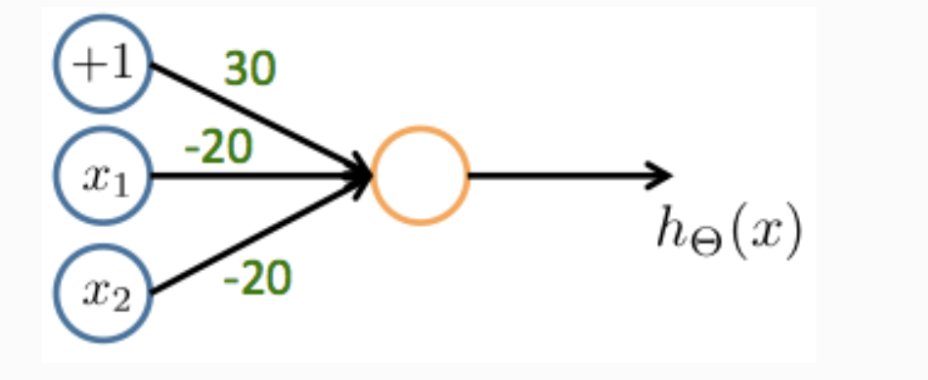• OR function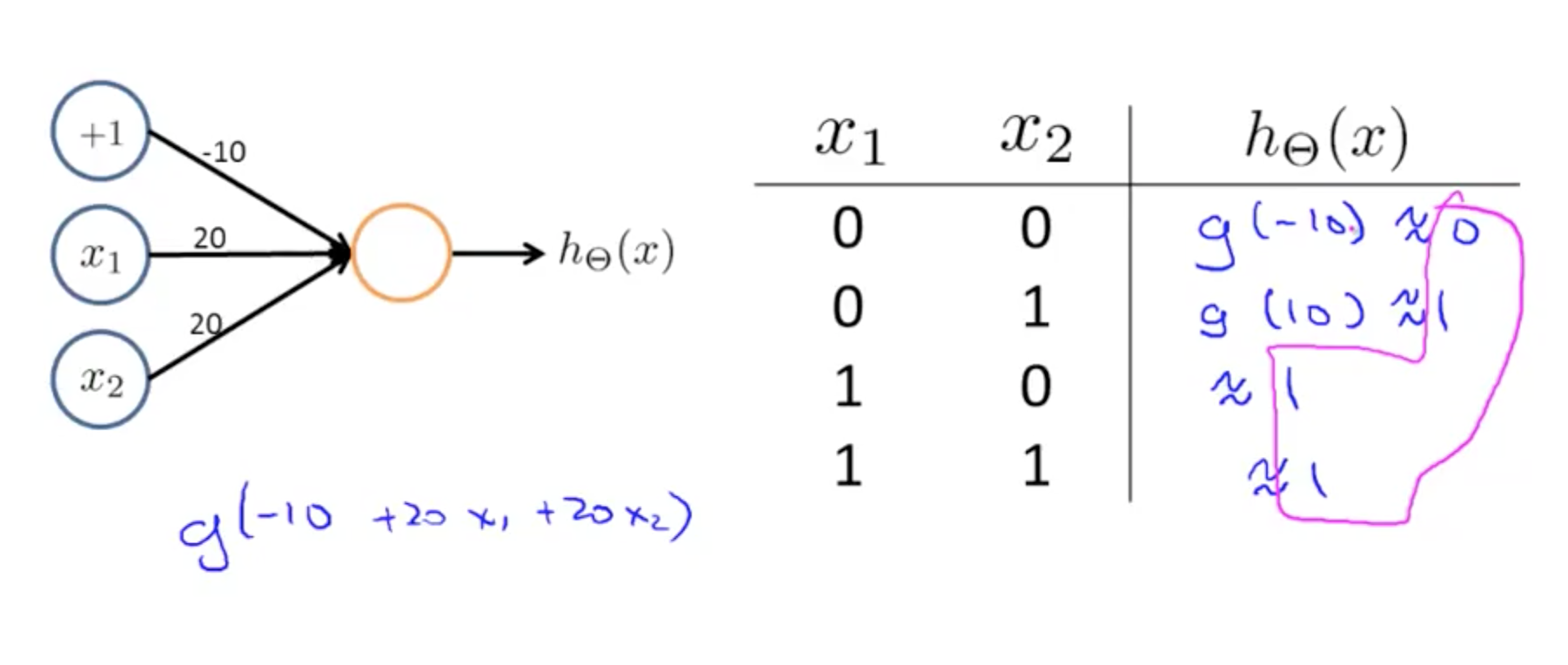### 2b. Examples and Intuitions II

• NOT function
•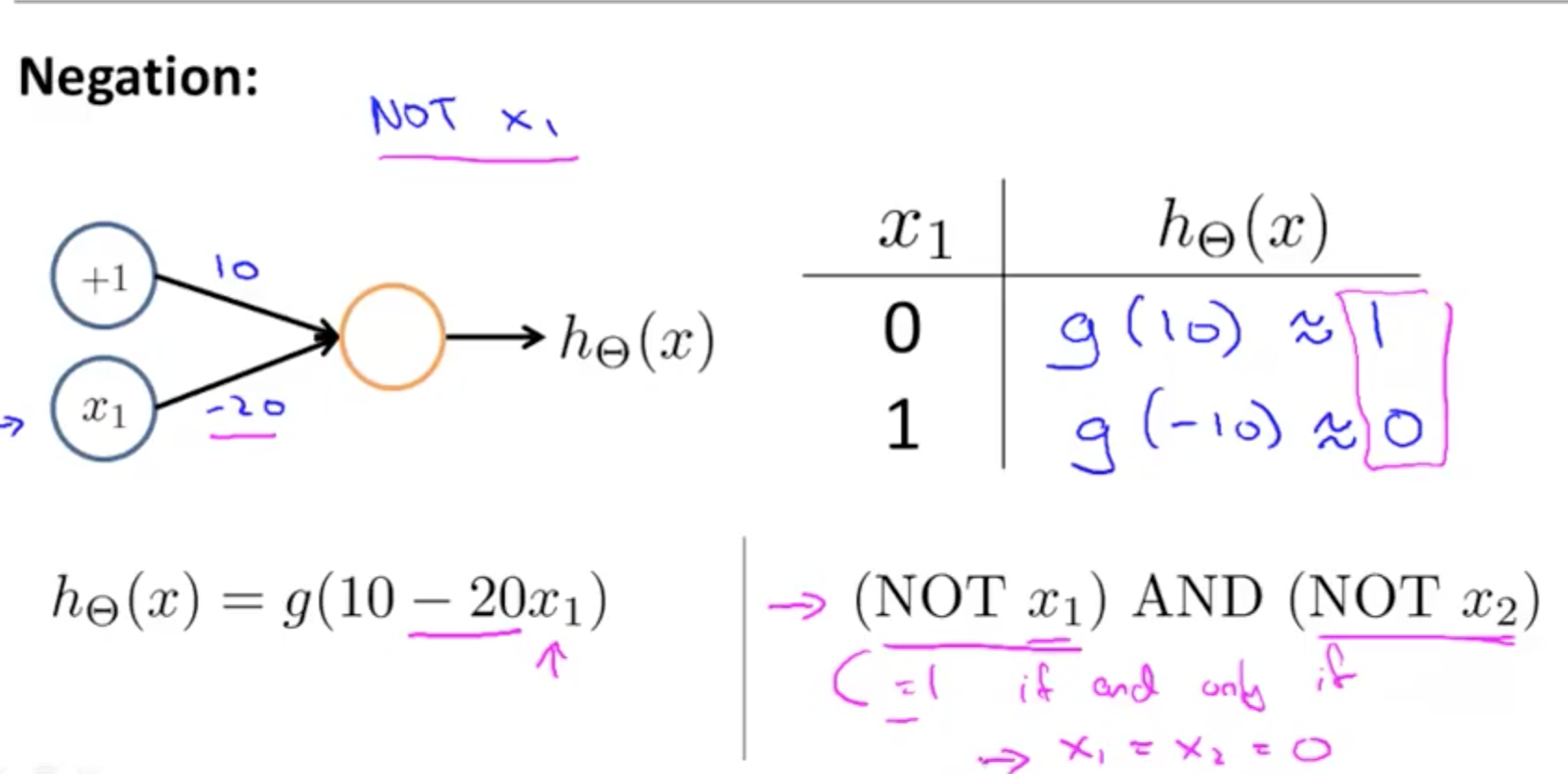• XNOR function
• NOT XOR
• NOT an exclusive or
• Hence we would want
• AND
• Neither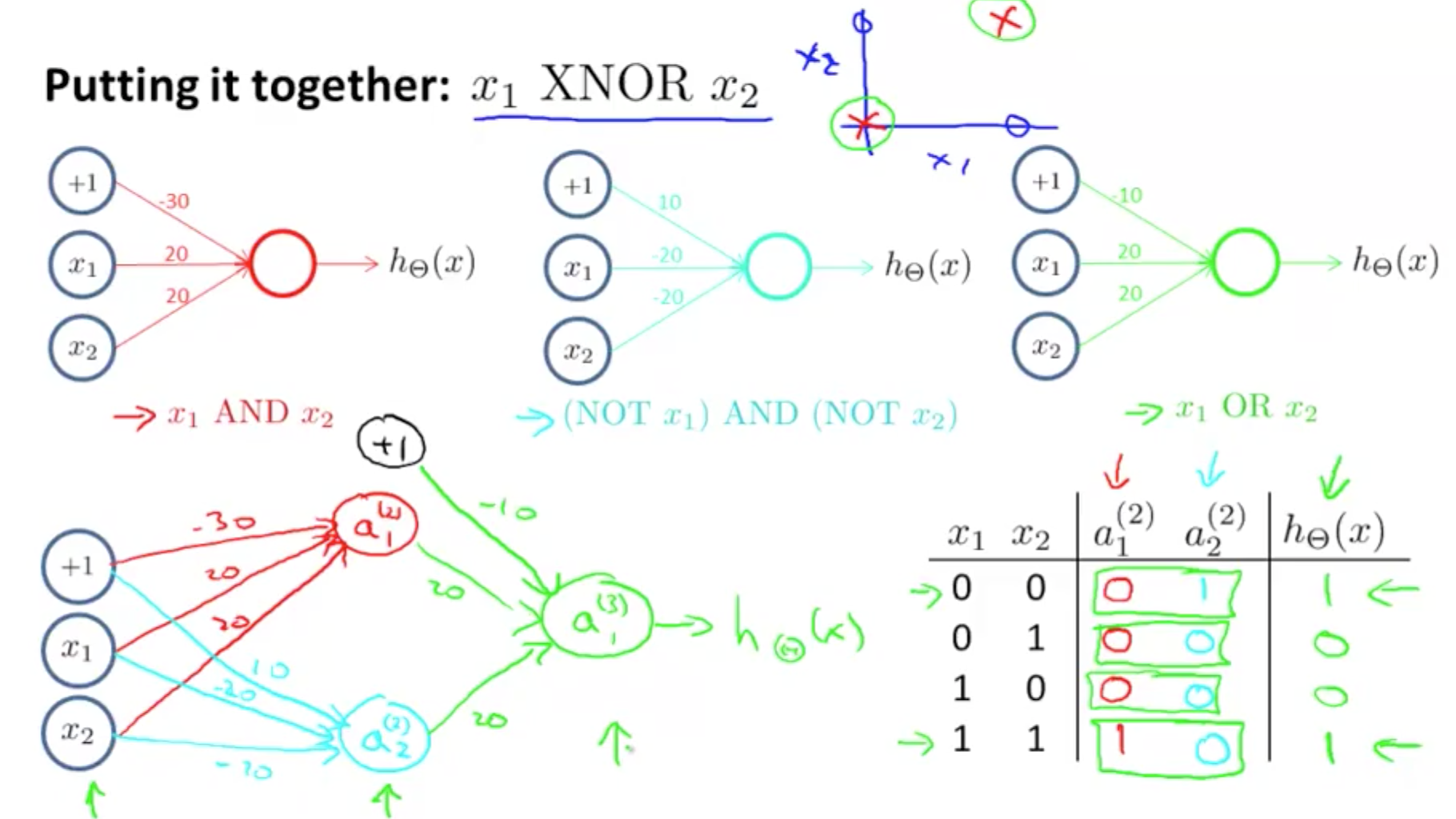### 2c. Multi-class Classification

• Example: identify 4 classes
• You would want a 4 x 1 vector for h_theta(X)
• 4 logistic regression classifiers in the output layer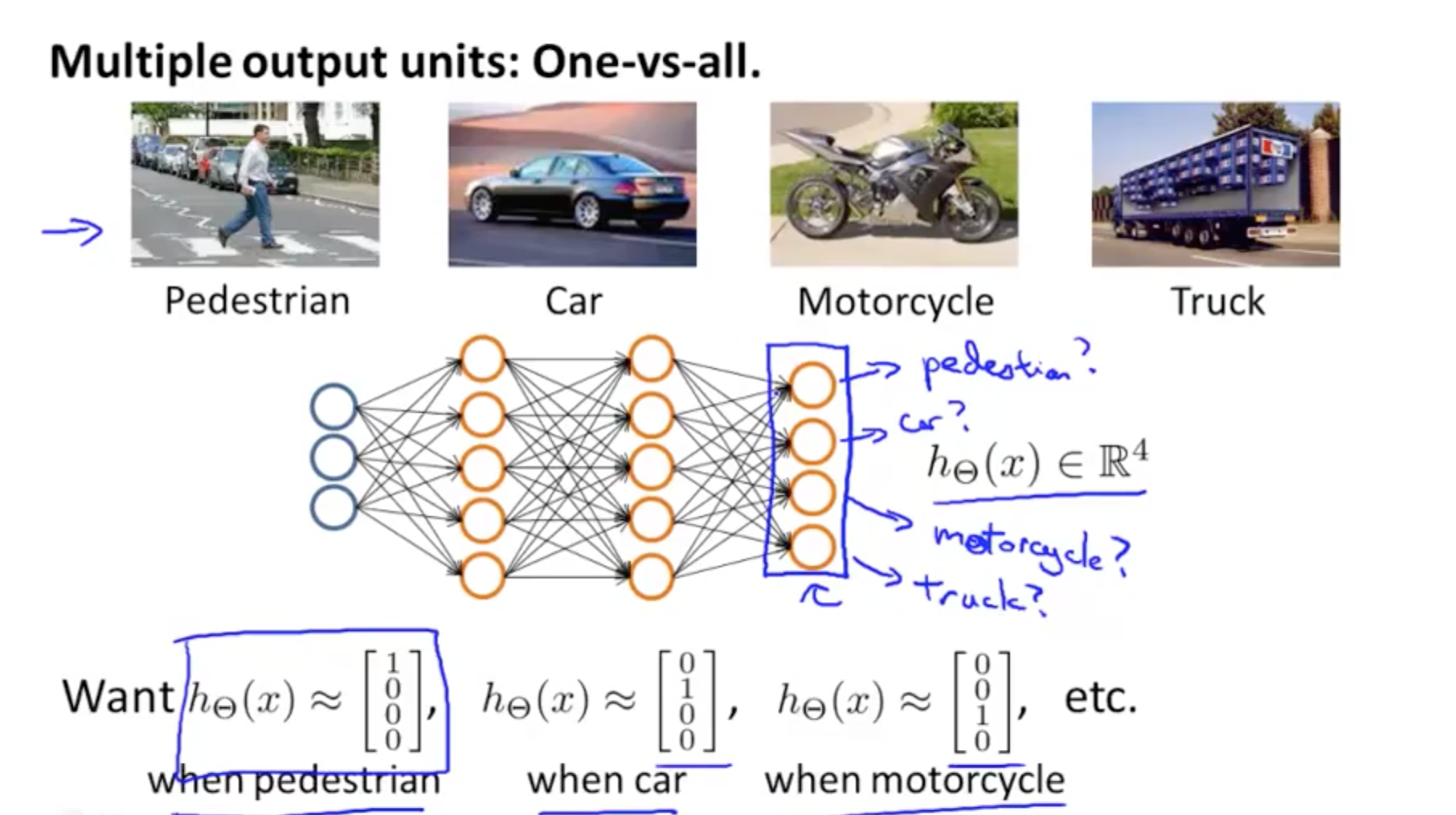• There will be 4 output
• y would be a 4 x 1 vector instead of an integer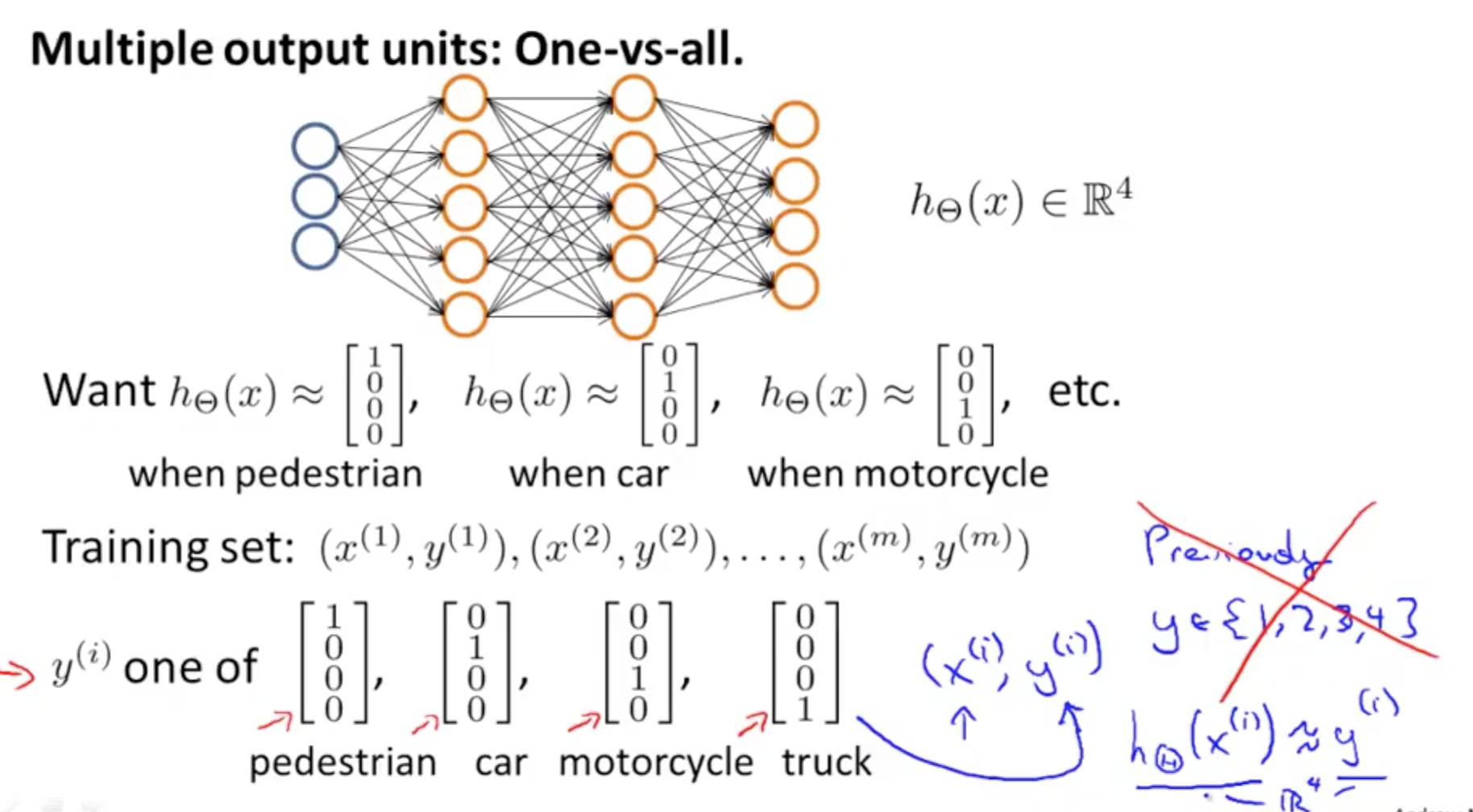Tags: